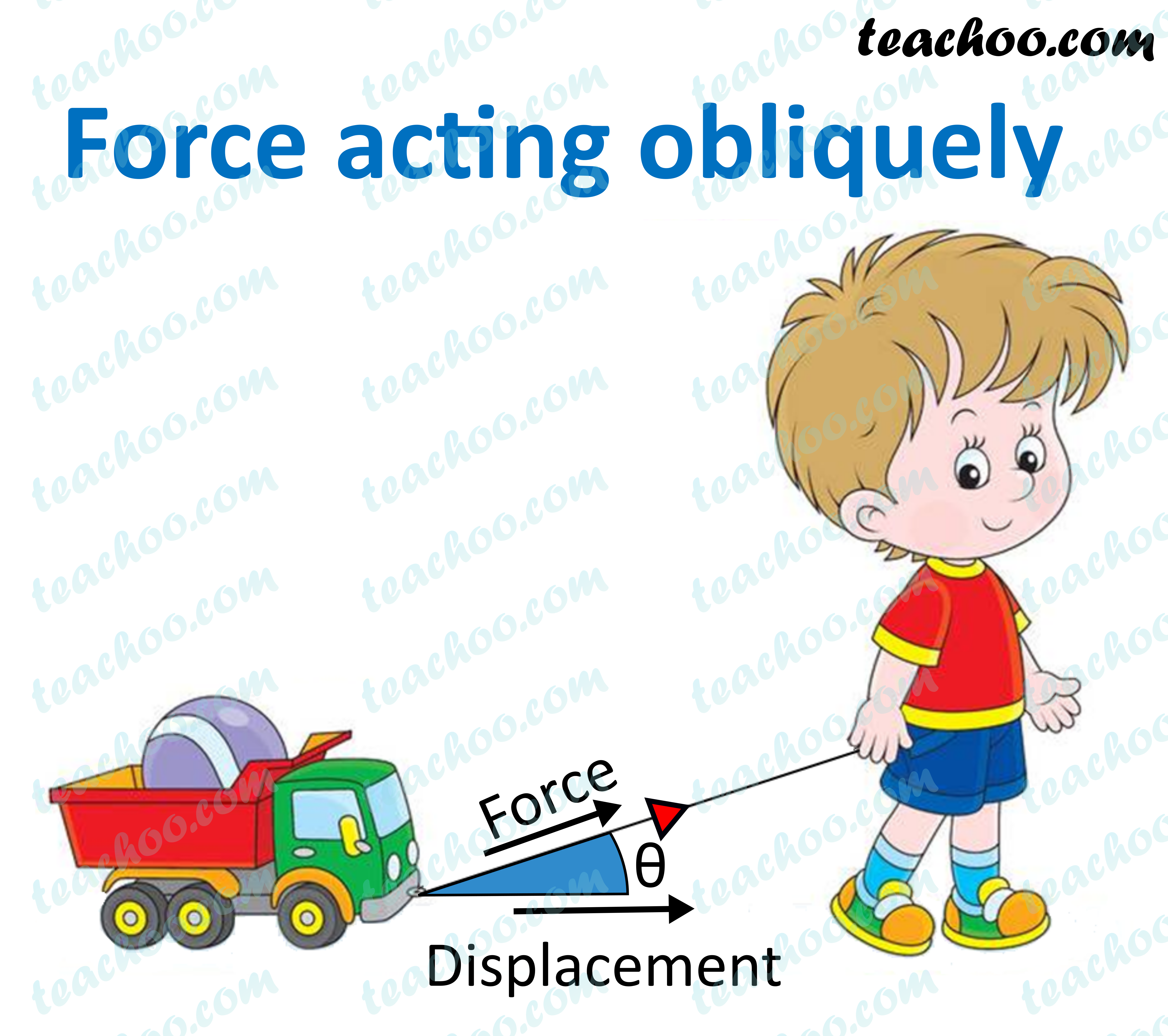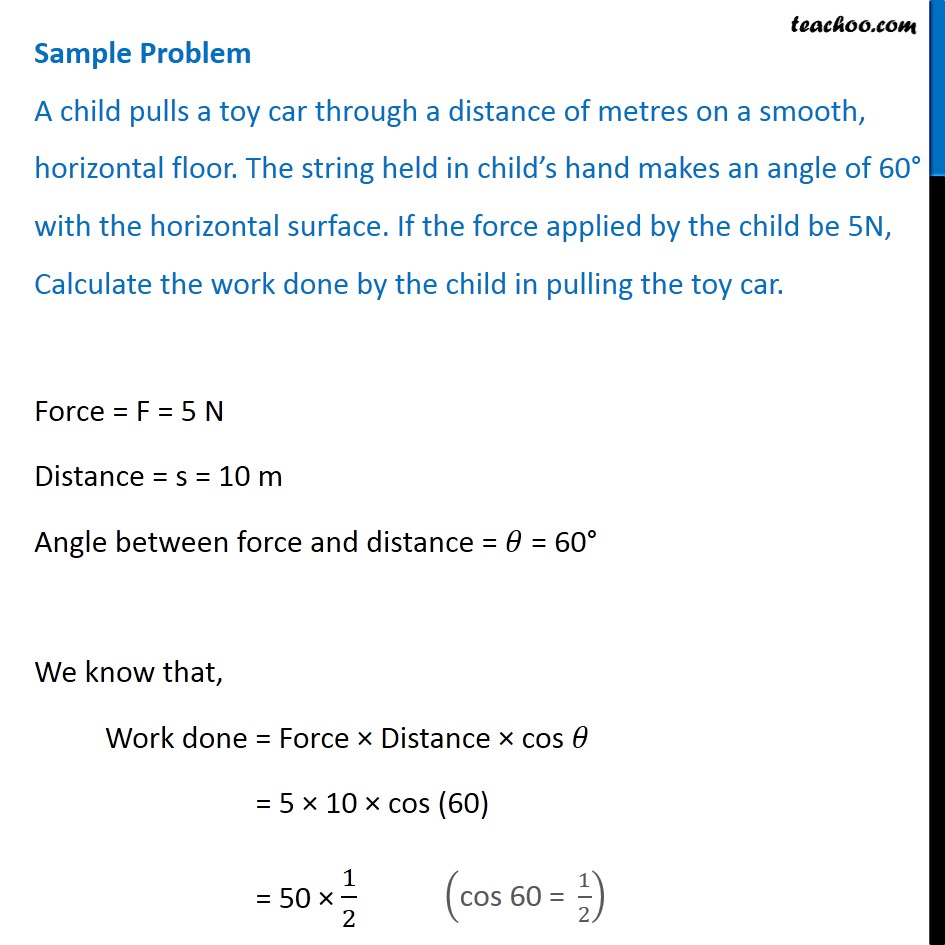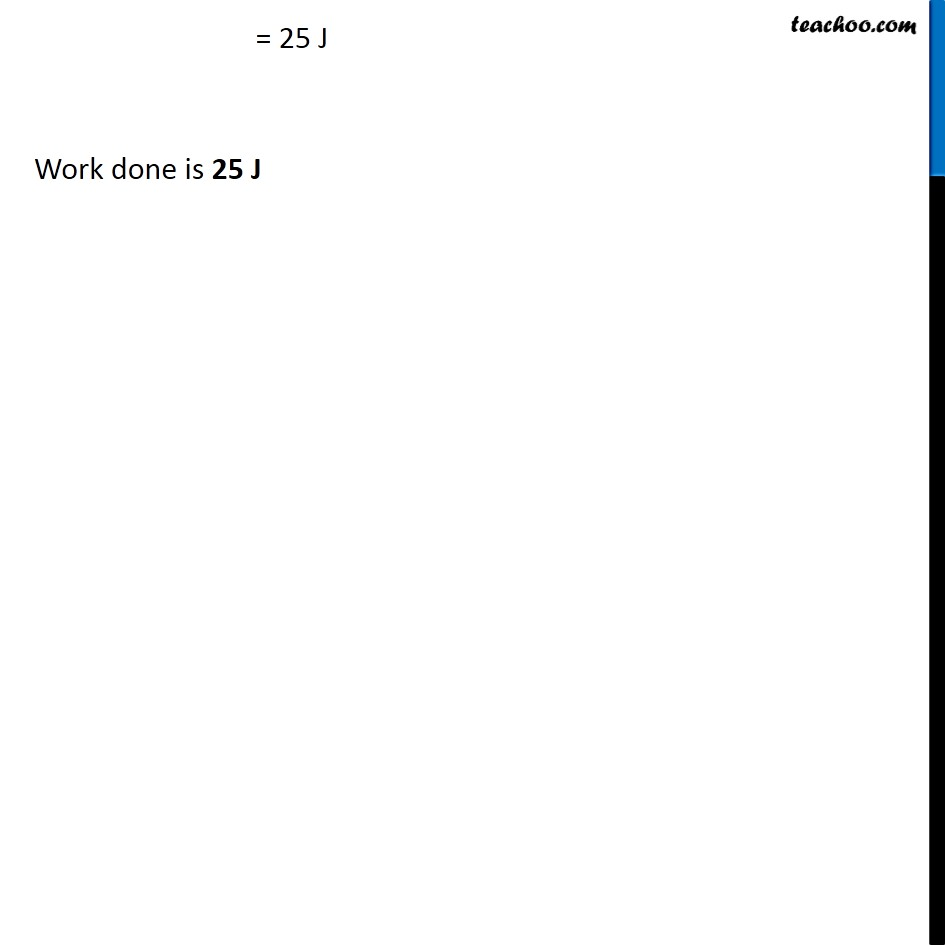Concepts

Class 9
Chapter 11 Class 9 - Work and Energy

## What is the meaning of 'Force Acting Obliquely'?

Sometimes, movement of body is not in direction of force applied

F force is applied in different direction but body moves in a different direction

This is called force acting obliquely or force acting indirectly

In this case, there is an angle between direction of force applied and direction of movement of object.

Example

When a child pulls a toy car with a string

force applied is in different direction but car moves forward in different direction (making an angle)## How to calculate work done in case of Force Acting Obliquely

In this case, we cannot use the formula

Work = Force × Distance

In this case, we cannot use Force because force is not in direction of movement of object

In this case, we cannot use the formula

So our formula becomes

Work = Force × Distance × cos θ

where θ is the angle between distance and force

## Common Values of Cos θ

Some common values of cos θ for angles 0, 30, 45, 60, 90 are

 DEGREE COS THETA VALUE 30 Degree √3/2 60 Degree 1/2 90 Degree 0 180 Degree -1 45 Degree 1/√2 0 Degree 1

## Questions

###(adsbygoogle = window.adsbygoogle || []).push({});Learn in your speed, with individual attention - Teachoo Maths 1-on-1 Class

### Transcript

Question A child pulls a toy car through a distance of metres on a smooth, horizontal floor. The string held in child’s hand makes an angle of 60° with the horizontal surface. If the force applied by the child be 5N, Calculate the work done by the child in pulling the toy car. Force = F = 5 N Distance = s = 10 m Angle between force and distance = 𝜃 = 60° We know that, Work done = Force × Distance × cos 𝜃 = 5 × 10 × cos (60) = 50 × 1/2 ("cos 60 = " 1/2) = 25 J Work done is 25 J